﻿ 中国海洋大学食堂菜谱的优化模型研究 Study on Mathematical Model of the Optimization of the Menu of OUC

Vol.07 No.02(2018), Article ID:23869,11 pages
10.12677/AAM.2018.72024

Study on Mathematical Model of the Optimization of the Menu of OUC

Jian Huang, Wangning Gao, Xiangdong Xie, Wenxuan Han, Zhihang Wang, Xiang Gao*

School of Mathematical Sciences, Ocean University of China, Qingdao ShandongReceived: Feb. 7th, 2018; accepted: Feb. 21st, 2018; published: Feb. 27th, 2018ABSTRACT

By using price and satisfactions of student we make a double objective integer linear programming model. At the last we add a fuzzy evaluation system to the model. This menu optimization model can solve nutritional intake problem and the problem of the waste of leftovers.

Keywords:Menu Optimization, Double Objective Linear Programming, Fuzzy Evaluation, MATLAB1. 引言

2. 问题的提出

3. 问题的分析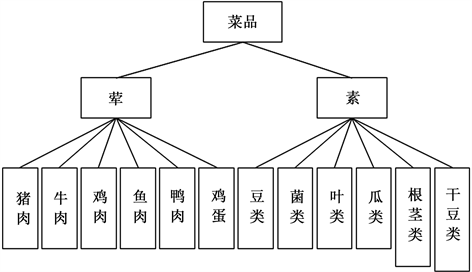Figure 1. Dishes hierarchy diagram

4. 模型的假设

1) 假设学生一天吃三顿饭、只打4份菜且都在同一窗口打饭，米饭摄入为400 g；

2) 假设每天到窗口A就餐人数是固定的，设为a；

3) 假设窗口A的菜品种类固定，每天所用食材固定，设为b；

4) 假设学生只存在营养不足问题，营养只来源于食材与米饭且不考虑营养过剩的情况；

5) 假设早饭的营养摄入量为一天所需营养的30%。

5. 模型的建立与优化

5.1. 模型的建立

$\mathrm{min}{z}_{1}={a}_{1}{q}_{1}{y}_{1}+{a}_{2}{q}_{2}{y}_{2}+\cdots +{a}_{n}{q}_{n}{y}_{n}$ (1)

$\mathrm{max}{z}_{2}={a}_{1}{p}_{1}{y}_{1}+{a}_{2}{p}_{2}{y}_{2}+\cdots +{a}_{n}{p}_{n}{y}_{n}$ (2)

PS: Men and women have different demand for iron and vitamin B1, B2, and take the average 注：铁、维生素b1、b2男女略有差异，这里取其均值

$\left\{\begin{array}{l}75×0.7-2.6×4\le 4m{x}_{1}\hfill \\ 50×0.7-0.3×4\le 4m{x}_{2}\hfill \\ 30×0.7-0.2×4\le 4m{x}_{3}\hfill \\ 1.35×0.7-0.02×4\le 4m{x}_{4}\hfill \\ 1.3×0.7-0.03×4\le 4m{x}_{5}\hfill \\ 800×0.7-7×4\le 4m{x}_{6}\hfill \\ 17.5×0.7-2.2×4\le 4m{x}_{7}\hfill \\ 15.5×0.7-1.36×4\le 4m{x}_{8}\hfill \\ m={a}_{1}{y}_{1}+{a}_{2}{y}_{2}+\cdots +{a}_{n}{y}_{n}\hfill \end{array}$

${y}_{n}$ 为菜品的种类

$m$ 为所选菜的总质量

${q}_{n}$ 为菜品 ${y}_{n}$ 的价格

${p}_{n}$ 为菜品 ${y}_{n}$ 的满意度

${a}_{n}$ 为一天中打到菜品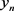的次数，其中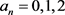5.2. 模型的优化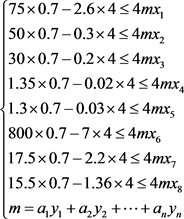6. 模型的求解

6.1. 窗口菜品营养元素计算

6.2. 窗口最优菜品组合计算

x2 = [35.4 5.65 14.92 7.392 0.2 1.5 18.6 10.801]

x3 = [0 0.35 0.72 0.3960 2.1 0 0.6 0.639]

x4 = [0.07 0.165 0.142 0.2409 0.05 0.01 0.155 0.0851]

x5 = [0.07 0.165 0.082 0.2211 0.17 0.07 0.095 0.0748]

x6 = [37 231.5 25.8 305.91 29 6 22.5 26.59]

x7 = [1.7 1.25 1.48 1.98 1.5 4.1 1.5 0.89]

x8 = [1.1 0.54 0.962 0.7425 0.54 1.17 1.145 1.0305]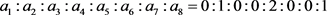Table 2. All kinds of nutrient elements in 100 g materialTable 3. All kinds of nutrient elements in the menu of window A

6.3. 窗口菜品制作数量计算

7. 模型的检验与评价

7.1. 建立模糊综合评价体系

1) 由之前讨论的因素作为菜谱模型合理性的因素集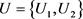，其中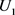表示菜品的价格，表示菜品的满意度.

2) 建立评价对象的等级集合V

3) 对于菜品价格的评价，V = {合理，一般，不合理}

4) 对于菜品满意度的评价，V = {满意，一般，不满意}Table 4. The price of the dishes in window A (Unit: Yuan)Table 5. The satisfaction of the dishes in window A (Range: 1~10)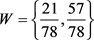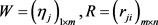7.2. 对食堂原菜谱建立隶属矩阵7.3. 模型求解计算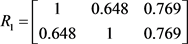7.4. 模糊评判集检验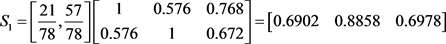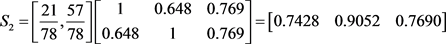8. 模型的应用与推广

Study on Mathematical Model of the Optimization of the Menu of OUC[J]. 应用数学进展, 2018, 07(02): 195-205. http://dx.doi.org/10.12677/AAM.2018.72024

1. 1. 胡敏. 新编营养师手册(第三版) [M]. 化学工业出版社, 2016.

2. 2. 龚勋. 邢涛. 菜谱大全[M]. 云南: 云南教育出版社, 2010.

3. 3. 杜栋, 庞华庆, 吴英. 现代综合评价方法与案例精选[M]. 北京: 清华大学出版社, 2008.

4. 4. 姜启源, 谢金星, 叶俊. 数学模型(第四版) [M]. 高等教育出版社, 2017.

5. 5. 武松, 潘发明. SPSS统计分析大全[M]. 北京: 清华大学出版社, 2014.

model:

!Lingo 求解双目标多元线性整数规划;

min=21/78*(x1*6+x2*2+x3*7+x4*3+x5*1.6+x6*6+x7*7+x8*2.4)-57/78*(x1*6.18+x2*5.77+x3*6.96+x4*5.72+x5*5.68+x6*7.14+x7*6.84+x8*5.9);

16.7*x1+6.6*x2+6.42*x3+9.075*x4+2.5*x5+15*x6+7.55*x7+4.822*x8>=(75*0.7-4*2.6)/4;

35.4*x1+5.65*x2+14.92*x3+7.392*x4+0.2*x5+1.5*x6+18.6*x7+10.801*x8>=(50*0.7-4*0.3)/4;

0.35*x2+0.72*x3+0.396*x4+2.1*x5+0.6*x7+0.639*x8>=(30*0.7-4*0.2)/4;

0.07*x1+0.165*x2+0.141*x3+0.2409*x4+0.05*x5+0.01*x6+0.155*x7+0.0851*x8>=(1.35*0.7-4*0.02)/4;

0.07*x1+0.165*x2+0.082*x3+0.2211*x4+0.17*x5+0.07*x6+0.095*x7+0.0748*x8>=(1.3*0.7-4*0.03)/4;

37*x1+231.5*x2+25.8*x3+305.91*x4+29*x5+6*x6+22.5*x7+26.59*x8>=(800*0.7-4*7)/4;

1.7*x1+1.25*x2+1.48*x3+1.98*x4+1.5*x5+4.1*x6+1.5*x7+0.89*x8>=(17.5*0.7-4*2.2)/4;

1.1*x1+0.54*x2+0.962*x3+0.7425*x4+0.54*x5+1.17*x6+1.145*x7+1.0305*x8>=(15.5*0.7-4*1.36)/4;

x1+x2+x3+x4+x5+x6+x7+x8=4;

@bnd(0,x1,2);

@bnd(0,x2,2);

@bnd(0, x3,2);

@bnd(0,x4,2);

@bnd(0,x5,2);

@bnd(0, x6,2);

@bnd(0,x7,2);

@bnd(0,x8,2);

@gin(x1);

@gin(x2);

@gin(x3);

@gin(x4);

@gin(x5);

@gin(x6);

@gin(x7);

@gin(x8);

function B=fenlei(A);

A=input('请输入菜品材料比例')%以矩阵表示，小数表示所占比例，无该种材料则为0，牛肉，猪肉，鱼肉，鸡肉，鸭肉，豆类，根茎类，菌类，叶类，干豆类，瓜果类，鸡蛋的顺序

G=[18.1,13.2,16.6,16.7,15,2.5,1.9,1.9,1.4,0.4,8.1,12.8

13.4,37,5.2,35.4,1.5,0.2,0.2,0.3,0.1,0.2,3.7,11.1

0,0,0,0,0,2.1,1.2,2.3,0.9,0.7,0.4,0

8,6,38,37,6,29,39,5,35,19,164,444

3.2,1.6,0.8,1.7,4.1,1.5,1.4,1,0.6,0.2,1.9,2.3

3.67,2.06,0.87,1.1,1.17,0.54,0.23,0.61,0.61,0.07,1.11,1.01

0.03,0.22,0.04,0.07,0.01,0.05,0.09,0.06,0.03,0.01,0.03,0.32

0.11,0.16,0.11,0.07,0.07,0.17,0.03,0.16,0.04,0.01,0.03,0.32];

M=zeros(8,12);

if any(abs(A)>0);

i=1;

while i<=12

if A(i)>0;

M(:,i)=G(:,i);

else

M(:,i)=[0 0 0 0 0 0 0 0]'

end

i=i+1;

end

else

i=1;

while i<=12

M(:,i)=[0 0 0 0 0 0 0 0]';

i=i+1;

end

end

M%输出顺序为蛋白质，脂肪，膳食纤维，维生素b1，维生素b2，钙，铁，锌

function D=goumaiqushishu(C,E);%

C=input('请输入菜品满意度');

E=input('请输入菜品价格'); %菜品满意度和价格都已矩阵的形式输入，例如3道菜则[1 2 3]

D=57/78*C-21/78*E

NOTES



*通讯作者。